Search by Topic

Resources tagged with Squares similar to Sorting Logic Blocks:

Filter by: Content type:
Age range:
Challenge level:

There are 38 results

Broad Topics > Angles, Polygons, and Geometrical Proof > Squares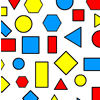Sorting Logic Blocks

Age 5 to 11 Challenge Level:

This interactivity allows you to sort logic blocks by dragging their images.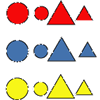Logic Block Collections

Age 5 to 7 Challenge Level:

What do you think is the same about these two Logic Blocks? What others do you think go with them in the set?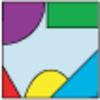Jig Shapes

Age 5 to 11 Challenge Level:

Can you each work out what shape you have part of on your card? What will the rest of it look like?Data Shapes

Age 5 to 7 Challenge Level:

Sara and Will were sorting some pictures of shapes on cards. "I'll collect the circles," said Sara. "I'll take the red ones," answered Will. Can you see any cards they would both want?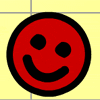Complete the Square

Age 5 to 7 Challenge Level:

Complete the squares - but be warned some are trickier than they look!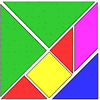Tangrams

Age 5 to 7 Challenge Level:

Can you make five differently sized squares from the tangram pieces?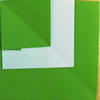Paper Patchwork 1

Age 5 to 7 Challenge Level:

Can you work out what shape is made when this piece of paper is folded up using the crease pattern shown?Halving

Age 5 to 7 Challenge Level:

These pictures show squares split into halves. Can you find other ways?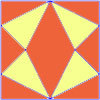Fraction Fascination

Age 7 to 11 Challenge Level:

This problem challenges you to work out what fraction of the whole area of these pictures is taken up by various shapes.Cut and Make

Age 7 to 11 Challenge Level:

Cut a square of paper into three pieces as shown. Now,can you use the 3 pieces to make a large triangle, a parallelogram and the square again?Inside Seven Squares

Age 7 to 11 Challenge Level:

What is the total area of the four outside triangles which are outlined in red in this arrangement of squares inside each other?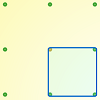Peg and Pin Boards

Age 5 to 11

This article for teachers suggests activities based on pegboards, from pattern generation to finding all possible triangles, for example.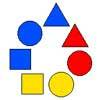Chain of Changes

Age 5 to 7 Challenge Level:

Arrange the shapes in a line so that you change either colour or shape in the next piece along. Can you find several ways to start with a blue triangle and end with a red circle?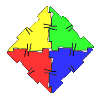Polydron

Age 7 to 11 Challenge Level:

This activity investigates how you might make squares and pentominoes from Polydron.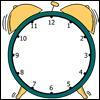A Square in a Circle

Age 7 to 11 Challenge Level:

What shape has Harry drawn on this clock face? Can you find its area? What is the largest number of square tiles that could cover this area?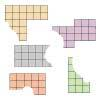Torn Shapes

Age 7 to 11 Challenge Level:

These rectangles have been torn. How many squares did each one have inside it before it was ripped?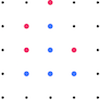Square Surprise

Age 5 to 11 Challenge Level:

Why do you think that the red player chose that particular dot in this game of Seeing Squares?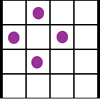Square Corners

Age 7 to 11 Challenge Level:

What is the greatest number of counters you can place on the grid below without four of them lying at the corners of a square?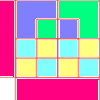Diagrams

Age 7 to 11 Challenge Level:

A group activity using visualisation of squares and triangles.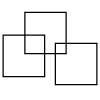Three Squares

Age 5 to 11 Challenge Level:

What is the greatest number of squares you can make by overlapping three squares?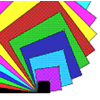Overlapping Squares

Age 7 to 11 Challenge Level:

Have a good look at these images. Can you describe what is happening? There are plenty more images like this on NRICH's Exploring Squares CD.Transformations on a Pegboard

Age 7 to 11 Challenge Level:

How would you move the bands on the pegboard to alter these shapes?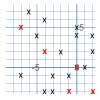Ten Hidden Squares

Age 7 to 11 Challenge Level:

These points all mark the vertices (corners) of ten hidden squares. Can you find the 10 hidden squares?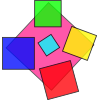Making Squares

Age 7 to 11

Investigate all the different squares you can make on this 5 by 5 grid by making your starting side go from the bottom left hand point. Can you find out the areas of all these squares?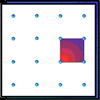Geoboards

Age 7 to 11 Challenge Level:

This practical challenge invites you to investigate the different squares you can make on a square geoboard or pegboard.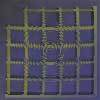My New Patio

Age 7 to 11 Challenge Level:

What is the smallest number of tiles needed to tile this patio? Can you investigate patios of different sizes?Fitted

Age 7 to 11 Challenge Level:

Nine squares with side lengths 1, 4, 7, 8, 9, 10, 14, 15, and 18 cm can be fitted together to form a rectangle. What are the dimensions of the rectangle?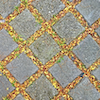Seeing Squares

Age 5 to 11 Challenge Level:

Players take it in turns to choose a dot on the grid. The winner is the first to have four dots that can be joined to form a square.Great Squares

Age 7 to 11 Challenge Level:

Investigate how this pattern of squares continues. You could measure lengths, areas and angles.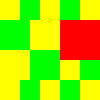Tiles on a Patio

Age 7 to 11 Challenge Level:

How many ways can you find of tiling the square patio, using square tiles of different sizes?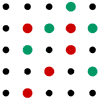Seeing Squares for Two

Age 5 to 11 Challenge Level:

Seeing Squares game for an adult and child. Can you come up with a way of always winning this game?Rope Mat

Age 7 to 11 Challenge Level:

How many centimetres of rope will I need to make another mat just like the one I have here?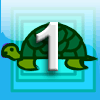First Forward Into Logo 1: Square Five

Age 7 to 16 Challenge Level:

A Short introduction to using Logo. This is the first in a twelve part series.LOGO Challenge - the Humble Square

Age 7 to 16 Challenge Level:

Look at how the pattern is built up - in that way you will know how to break the final pattern down into more manageable pieces.Logo Challenge 3 - Star Square

Age 7 to 16 Challenge Level:

Creating designs with squares - using the REPEAT command in LOGO. This requires some careful thought on anglesZooming in on the Squares

Age 7 to 14

Start with a large square, join the midpoints of its sides, you'll see four right angled triangles. Remove these triangles, a second square is left. Repeat the operation. What happens?Eight Hidden Squares

Age 7 to 14 Challenge Level:

On the graph there are 28 marked points. These points all mark the vertices (corners) of eight hidden squares. Can you find the eight hidden squares?Baravelle

Age 7 to 16 Challenge Level:

What can you see? What do you notice? What questions can you ask?